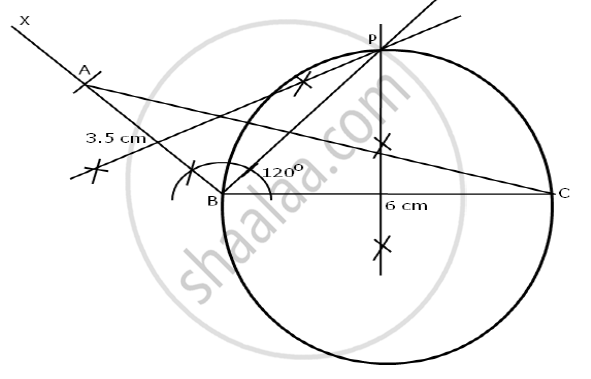Share

# Using a Ruler and Compasses Only: 1) Construct a Triangle Abc with the Following Data: Ab = 3.5 Cm, Bc = 6 Cm and Abc = 120° 2) In the Same Diagram, Draw a Circle with Bc as Diameter. Find a Point P on the Circumference of the Circle Which is Equidistant from Ab and Bc. 3) Measure ∠Bcp. - ICSE Class 10 - Mathematics

ConceptCircumscribing and Inscribing a Circle on a Triangle

#### Question

Using a ruler and compasses only:

1) Construct a triangle ABC with the following data: AB = 3.5 cm, BC = 6 cm and ABC = 120°

2) In the same diagram, draw a circle with BC as diameter. Find a point P on the circumference of the circle which is equidistant from AB and BC.

3) Measure ∠BCP.

#### Solution

1) Steps of constructions:

• Draw a line segment BC = 6 cm.
• At B, draw a ray BX making an angle of 120o with BC.
• From the point, B cut an arc of radius 3.5 cm to meet ray BX at A.
• Join AC.

ABC is the required triangle.

2)

Bisect BC and draw a circle with BC as diameter.

Draw perpendicular bisectors of AB. Let the two bisectors meet the ray of angle bisector of ∠ABC at point P. P is equidistant from AB and BC.

3) On measuring ∠BCP = 30°Is there an error in this question or solution?

#### Video TutorialsVIEW ALL 

Solution Using a Ruler and Compasses Only: 1) Construct a Triangle Abc with the Following Data: Ab = 3.5 Cm, Bc = 6 Cm and Abc = 120° 2) In the Same Diagram, Draw a Circle with Bc as Diameter. Find a Point P on the Circumference of the Circle Which is Equidistant from Ab and Bc. 3) Measure ∠Bcp. Concept: Circumscribing and Inscribing a Circle on a Triangle.
S